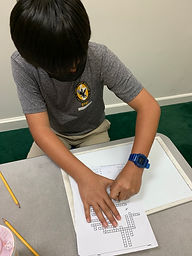Ms. Kayla

Target 1​

Lesson Type:

Review

Number Operation

:

Computation

Multiply and divide fractional numbers to any place-value.

1:

Utilize the standard algorithm to divide fractions.

2:

With two fractions, find the reciprocal of the 2nd fraction, replace the ÷ with x, multiply numerators and denominators, simplify.

3:

With a whole number by a fraction, convert the whole number to an improper fraction, find the reciprocal of the 2nd fraction, replace the ÷ with x, multiply numerators and denominators, simplify.

4:

With a fraction by a whole number, convert the whole number to improper fraction, find the reciprocal of the 2nd number, replace the ÷ with x, multiply the numerators and denominators, simplify.

6th

Vocabulary:

Inverse, Mixed Number, Improper Fraction, Convert, Reduce, Simplest Form

Activities:

1. Students needed to convert the mixed numbers into an improper fraction to continue multiplying or dividing.

2. Students used the reciprocal of the second fraction when they inversed the division operation to solve the problem.

3. Students reviewed reducing a fraction to its lowest term, but also learned how to reduce a fraction through cross cancellation.Home Exploration

Challenge Problem:

4 and 4/5 divided by 4/12

Guiding Questions:

14 and 2/5Absent Students:

Target 2

:

1:

Identify the correct arithmetic process based on the information presented in word problems.

2:

Find and use the needed information in a word problem to solve.

7th

Vocabulary:

Unit Rate, Quantity, Covert, Unit Price, Simplify

Activities:

1. Students solved word problems invovling unit rates in real world situations (i.e., grocery store items, snow/rain fall, race, etc.).

2. Students needed to determine the most efficient method to effectively solve the problems (i.e., repeated addition, multiplication, division, etc.).

3. Problems included unit rates for unit prices, fraction quantities, and ratios.Home Exploration

Tom needs to buy bags of chips. He has \$10 and a bag of chips is \$2.59 each. How many bags of chips can he buy? How much change should he get back?

Tom can buy 3 bags of chips and should get \$2.23 back.

Guiding Questions:Target 3

:

Vocabulary:

Activities:Home Exploration